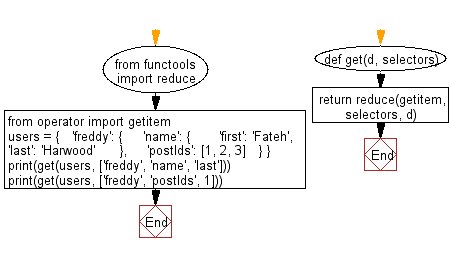﻿ Python: Retrieve the value of the nested key indicated by the given selector list from a dictionary or list - w3resource# Python: Retrieve the value of the nested key indicated by the given selector list from a dictionary or list

## Python List: Exercise - 225 with Solution

Write a Python program to retrieve the value of the nested key indicated by the given selector list from a dictionary or list.

• Use functools.reduce() to iterate over the selectors list.
• Apply operator.getitem() for each key in selectors, retrieving the value to be used as the iteratee for the next iteration.

Sample Solution:

Python Code:

``````from functools import reduce
from operator import getitem
def get(d, selectors):
return reduce(getitem, selectors, d)
users = {
'freddy': {
'name': {
'first': 'Fateh',
'last': 'Harwood'
},
'postIds': [1, 2, 3]
}
}
print(get(users, ['freddy', 'name', 'last']))
print(get(users, ['freddy', 'postIds', 1]))
```
```

Sample Output:

```Harwood
2
```

Flowchart:## Visualize Python code execution:

The following tool visualize what the computer is doing step-by-step as it executes the said program:

Python Code Editor:

Have another way to solve this solution? Contribute your code (and comments) through Disqus.

What is the difficulty level of this exercise?

Test your Python skills with w3resource's quiz

﻿

## Python: Tips of the Day

Floor Division:

When we speak of division we normally mean (/) float division operator, this will give a precise result in float format with decimals.

For a rounded integer result there is (//) floor division operator in Python. Floor division will only give integer results that are round numbers.

```print(1000 // 300)
print(1000 / 300)```

Output:

```3
3.3333333333333335```